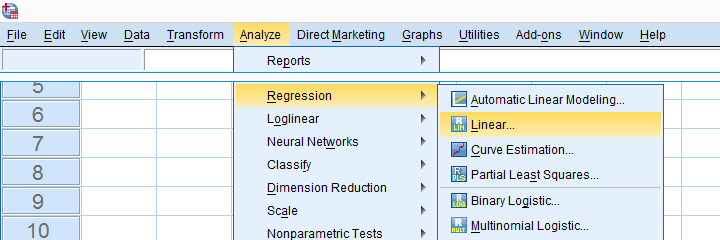SPSS TUTORIALS BASICS ANOVA REGRESSION FACTOR CORRELATION

# SPSS Regression Tutorials – Overview## Multiple Linear Regression Tutorials

### SPSS Multiple Linear Regression Example

This tutorial walks you through a complete multiple regression analysis in SPSS:

### SPSS Multiple Regression Analysis Tutorial

How to run multiple regression in SPSS the right way? This simple tutorial quickly walks you through the right steps in the right order. With superb illustrations and downloadable practice data file.

## Regression - Dummy Variables

### Creating Dummy Variables in SPSS

Quick guide on creating dummy variables in SPSS for categorical predictors in regression.

Intermediate tutorial with practice data, examples and a handy tool.

### SPSS Dummy Variable Regression Tutorial

How to run and interpret dummy variable regression in SPSS?

These 3 examples walk you through everything you need to know.

## Regression with Moderation Interaction

### How to Mean Center Predictors in SPSS?

For mean centering predictors in SPSS, just add their means to the data. Then simply subtract them from the original variables. With examples & practice data.

### SPSS Moderation Regression Tutorial

How to run a regression analysis with a moderation interaction effect? This SPSS example analysis walks you through step-by-step.

## SPSS Regression Tools

### SPSS – Create Dummy Variables Tool

Categorical variables can't readily be used as predictors in regression analysis; they must be split up into dichotomous variables known as “dummy variables”.

This tutorial offers a super easy tool for creating these.

### SPSS Mean Centering and Interaction Tool

"I'd like to mean center a lot of variables in order to compute interaction terms for a regression analysis. Is there an easy way to do this for many variables simultaneously?"

### SPSS Scatterplots & Fit Lines Tool

This simple SPSS tool creates one, many or all scatterplots among a set of variables.

Optionally, it adds (non)linear fit lines and regression tables as well.

## SPSS Stepwise Regression

### SPSS – Data Preparation for Regression

Before running SPSS stepwise regression, first just get a grip on your data. This tutorial walks you through the essential data checks.

### SPSS Stepwise Regression – Simple Tutorial

SPSS stepwise regression example. Easy-to-follow explanation of what and why with downloadable data file and annotated output.

### SPSS Stepwise Regression Tutorial II

SPSS stepwise regression analysis in normal language. With illustrations, downloadable practice data and syntax.

## SPSS Regression Tutorials - Other

### Multiple Linear Regression – What and Why?

Multiple regression is a statistical technique that aims to predict a variable of interest from several other variables. This tutorial explains multiple regression in normal language with many illustrations and examples.

### How to Draw a Regression Line in SPSS

This tutorial walks you through several simple options for creating scatterplots with regression lines.

We'll also cover linear and nonlinear regression lines for all cases or subgroups of cases.

## Simple Linear Regression Tutorials

### SPSS Simple Linear Regression Tutorial

SPSS Simple Linear Regression - Tutorial & Example

### Simple Linear Regression – Quick Introduction

This tutorial gently walks you through the basics of simple regression: b and beta coefficients, the intercept and r-square (adjusted). Get this right and you'll get it all right.

## Logistic Regression

### Logistic Regression

Logistic regression is a technique for predicting a dichotomous outcome variable from 1+ predictors.

This simple introduction quickly walks you through all logistic regression basics with a downloadable example analysis.

# Tell us what you think!

*Required field. Your comment will show up after approval from a moderator.

# THIS TUTORIAL HAS 8 COMMENTS:

•### By Chandrasekhar Subramanyam on May 10th, 2018

I used SPSS EXPERT Modeller with one dependent and four predictors.In output it says one Predictor.
How to identify which one is this?
How do we get the ARIMA coefficients

Prof S Chandrasekhar

•### By SIKANDAR ABDUL QADIR on August 7th, 2018

Ordinal logistic regression with Interaction terms interpretation.

•### By Ruben Geert van den Berg on August 7th, 2018

We're going to expand on and cover linear multiple regression with moderation interaction pretty soon. Ordinal logistic regression -unfortunately- is not on our agenda just yet. Sorry!

•### By chernet on August 7th, 2019

Wow! It's good job

•### By Ro Gumm on July 15th, 2020

Do you have a tutorial on how to do partial least square regression analysis?